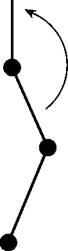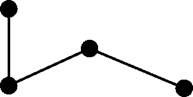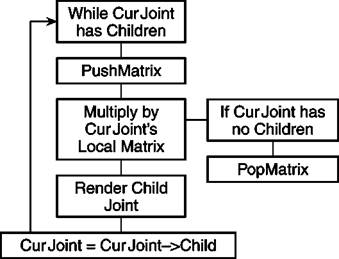# Taking Position

Using the information you have already read, you could try to imple­ment skeletal animation. However, you haven’t learned about how the parent joints actually affect the child joints. Simply using the keyframes would cause every joint to move independently of the rest, probably producing a strange, contorted mess.

This section talks about how to change this so that the joints work together. The first thing you do is build a transformation matrix for each point using the data from the current rotation and translation keyframes. A transformation matrix can be built by first generating the three rotation matrices and translation matrix as shown in Chapter 1. Multiplying the three together will produce a final transformation matrix. Alternatively you can use the SetRotation and SetTranslation functions in the matrix classes to avoid having to build and multiply the matrices yourself. This matrix is called the relative matrix.

Next, you need to calculate what is called the absolute matrix. The abso­lute matrix is the joint’s relative matrix multiplied by its parent’s abso­lute matrix. The absolute matrix tells you the joint’s absolute transfor­mation. This includes its relative transformations, as well as all of the transformations any joints before it in the hierarchy have made. This is what allows other joints to move as a result of moving a joint farther up in the line. Consider, for example, how your elbow moves when you move your shoulder. This begs the question: how do you calculate the

very first absolute matrix? Keep in mind that the root joint has no parent. Therefore, its absolute matrix is the same as its relative matrix.If you traverse down the joints in the right order, calculating the absolute matrices as you go, every joint will have its parent’s transfor­mations, and its parent’s parent’s transformations and so on. Figure 5.6 shows what happens when you take into account all previous transformations before transforming a joint.Rotating A joint with children affects the children, as well as the original joint

Figure 5.6 Traversing down the joints, taking into account all previous transformations. Notice that even though only one joint is told to move, the ones below it follow, much like moving your hip joint and having your knee and ankle follow as well.

What if you do not store the joint’s parent index, but rather the child indexes? This is no problem. The set of indexes you have access to right away depends on the model format. Some formats such as MS3D give you the parent index for each joint, whereas others give you the child indexes. Using child indexes requires a slightly different ap­proach than using parent indexes, but is really not any harder. You start at the root joint again. After calculating the root joint’s transfor­mation matrix, you push a new matrix onto the stack with a command such as glPushMatrix. This creates a new copy of the world matrix, which is the matrix that all geometry is transformed by before being dis­played. Now, multiply your new matrix by the root joint’s local matrix.

The resulting world matrix positions everything so when the next bone is drawn, the transformation of the parent joint is taken into consider­ation. For example, the hip of the character may be rotated a certain amount. Because the knee and ankle joints are children of the hip joint, they will also be rotated.

The drawing function is recursive so, as each joint is drawn, it calls the drawing functions of its children. Each child calls the rendering function of its children, and so on. Only when a terminal joint is reached (one with no children), is the matrix stack reset using a command such as glPopMatrix. For example, when drawing the leg of a character, new matrices can be pushed on the stack for the knee, angle, and foot joints. But when it is time to start on the arm, you want to pop back to the original position. Otherwise, whenever you moved the leg, the arm would move as well.

Figure 5.7 shows a diagram of a recursive rendering function.Figure 5.7 Rendering joints that are stored with child indexes instead of parent indexes